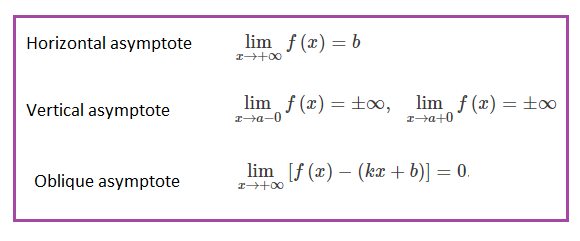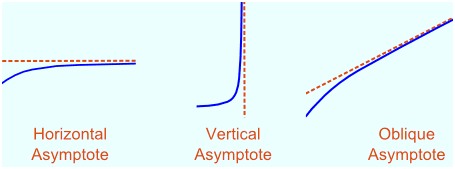# Asymptote Formula

In analytic geometry, an asymptote of a curve is a line such that the distance between the curve and the line approaches zero as they tend to infinity. In some contexts, such as algebraic geometry, an asymptote is defined as a line which is tangent to a curve at infinity. There are two types of asymptote: one is horizontal and other is vertical. Also, a special type of asymptote exists that is an oblique asymptote. Below mentioned are the asymptote formulas.This can be shown graphically as:### Solved Example

Question 1: Find the asymptotes for
$$\begin{array}{l}f(x) = \frac{2x^{2} + 2x}{x^{2}+1}\end{array}$$

Solution:

We can see at once that there are no vertical asymptotes as the denominator can never be zero.
$$\begin{array}{l}x^{2}\end{array}$$
+ 1 = 0
$$\begin{array}{l}x^{2}\end{array}$$
= –1 has no real solution.
Thus, this refers to the vertical asymptotes.

Now see what happens as x gets infinitely large:$\lim_{x\rightarrow\infty}\;\frac{2x^{2}+2x}{x^{2}+1}$

The method we have used before to solve this type of problem is to divide through by the highest power of x.

=

$$\begin{array}{l}\LARGE\lim_{x\rightarrow\infty}\;\frac{2x^{2}+2x}{x^{2}+1}\end{array}$$

$$\begin{array}{l}\LARGE \frac{\frac{2x^{2}}{x^{2}} + \frac{2x}{x^{2}}}{\frac{x^{2}}{x^{2}} + \frac{1}{x^{2}}}\end{array}$$

=

$$\begin{array}{l}\LARGE\frac{2+0}{1+0}\end{array}$$

=

$$\begin{array}{l}\LARGE2\end{array}$$

This is the horizontal asymptotes of the give function.

 More topics in Asymptote Formula Slant Asymptote Formula